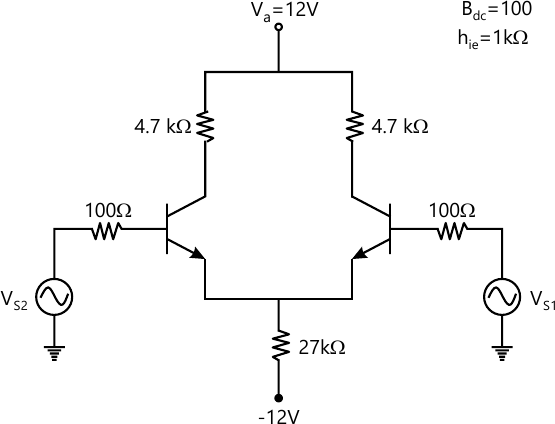MORE IN Analog & Digital Circuits
MU Information Technology (Semester 3)
Analog & Digital Circuits
May 2013
Total marks: --
Total time: --
INSTRUCTIONS
(1) Assume appropriate data and state your reasons
(2) Marks are given to the right of every question
(3) Draw neat diagrams wherever necessary

1 (a) Explain block diagram of op-amp
5 M
1 (b) Explain zero crossing detector
5 M
1 (c) Explain significance of CMRR for a differential amplifier
5 M
1 (d) Explain the basic principle of D to A converter
5 M

2 (a) Explain internal block diagram of astable multivibrator using IC 555 and explain the one application of it.
10 M
2 (b) For the differential amplifier find Ad, Ac, CMRR, Rin and Ro10 M

3 (a) Draw the transfer characteristics of an n-channel JFET with the help of Schockley's expression and explain its significance.
10 M
3 (b) Desing a wide bandpass filter for FL=1KHz and passband gain equal to 4. Also find quality factor.
10 M

4 (a) Draw the block diagram of an oscillator and expalin the Barkhausen conditions to obtain sustained oscilations.
10 M
4 (b) Design a stable multivibrator using 555 for duty cycle 75% and output frequency 5 kHz
10 M

5 (a) Explain the basic requirement for the instrumentation amplifier. Find the expression for output voltage using three op-amp.
10 M
5 (b) Explain the non-inverting schmitt trigger and give the schmitt trigger advantages over the conventional comparators.
10 M

6 (a) Design a voltage regulator using I2 723, Vo=5V, Io=50 mA, Isc=75 mA, vin=15V.
10 M
6 (b) Design a R-C phase shift oscillator for output frequency 1 KHz.
10 M

7 (a) Explain the averaging amplifier.
5 M
7 (b) Explain three terminal voltage regularor
5 M